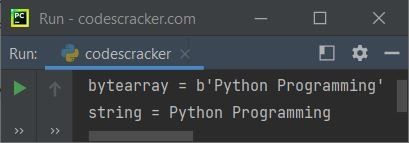# Python Convert bytearray to String

This article is created to cover a program in Python that converts a bytearray object to a string object. The program is created in a similar way, from bytes to string.

The question is, "Write a Python program to convert bytearray to string." The program given below is its answer:

```data = b'Python Programming'
print("bytearray =", data)
data = data.decode()
print("string =", data)```

The snapshot given below shows the sample output of the above Python program, demonstrating bytearray to string:In the above example, the first line initializes a bytearray object named data with the value 'Python Programming'. The b before the string indicates that it is a bytes object.

The second line uses the print() function to display the bytearray object. Since the object is a bytes object, the output will show a string of byte values enclosed in b''.

The third line assigns the value of data after decoding it to a new variable data which is now a string object.

The fourth line uses the print() function again to display the new string value of data. Since the decode() method is used to convert the bytes to a string, the output of the print statement will display the string value of 'Python Programming'.

## bytearray to String in Python

This program allows the user to enter the string. The string gets converted into bytearray with utf-8 encoding, and then the bytearray gets converted back to the string using the decode() method with utf-8 decoding.

```print("Enter the String: ", end="")
x = input()

print("\nThe string:")
print(x)

x = bytearray(x, "utf-8")
print("\nThe bytearray:")
print(x)

x = x.decode()
print("\nAgain the String:")
print(x)```

Here is its sample run with user input: codescracker dot comThe above example code prompts the user to enter a string and then reads it using the input() function. It then prints the input string, converts it to a bytearray using the bytearray() function with the encoding format "utf-8", and prints the resulting bytearray. The program then converts the bytearray back to a string using the decode() method with the "utf-8" encoding format and prints the resulting string.

If the bytearray object's data is encoded using another encoding, like utf-16, then we need to use the same decoding while converting the bytearray object to a string object using the decode() method.

Python Online Test

« Previous Program Next Program »